EXPERIMENT NO.1

TITLE: GENERATION OF ANY FOUR WAVEFORMS

THEORY:

1) SINUSOIDAL SIGNAL:

A sinusoidal is any function having the following form: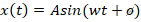Where t is the independent (real) variable and the fixed parameters A ,w, Ã¸ are all real constants . In audio applications we typically have,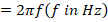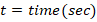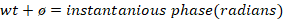The term â€œpeak amplitudeâ€ is often shortened to â€œamplitudeâ€, e.g., â€œthe amplitude of the tone was measured to be 5 Pascalâ€. Strictly speaking, however, the amplitude of signal x is its instantaneous valuex(t) at any time â€˜tâ€™. The peak amplitude A satisfies I x(t)<=AI. The â€œinstantaneous amplitudeâ€ or simply â€œ magnitudeâ€ of a signal x(t) is given by Ix(t)I, and the peak magnitude is the same thing as the peak amplitude.

EXPONENTIALS:

The conical form of an exponential function, as typically used in signal processing, isWhere â€™Ï„â€™ is called the time constant of the exponential. A is the peak amplitude, as before. The time constant is the time it takes to decay by, i.e.,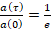A normalized exponential decay is depicted in fig. :

The decaying exponential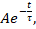normalized to unit amplitude).

WHY EXPONENTIALS ARE IMPORTANT:

Exponential decay occurs naturally when a quantity is decaying at a rate which proportional to how much is left. In nature all linear resonators such as musical instruments strings & woodwind bores, exhibit exponential decay in their response to a momentary excitation. As another example, reverberant energy in a room decays exponentially after the direct sounds stops. Essentially all undriven oscillations decay exponentially (provided they are linear and time invariant). Undriven means there is no ongoing source of driving energy, Examples of undriven oscillations include the variation of a tuning fork, struck or plucked strings, a marimba or xylophone bar, and so on. Example of driven oscillations includes horns, woodwinds, bowed strings, and voice. Driven oscillations must be periodic while undriven oscillations normally are not, except in idealized cases.

Exponential growth occurs when a quantity is increasing at the rate proportional to the current amount. Exponential growth is unstable since nothing can grow exponentially forever running into some kind of limit. Note that a positive time constant corresponds to exponential decay, while a negative time constant corresponds to exponential growth. In signal processing, we almost always deal exclusively with exponential decay (positive time constant).

Exponential growth and decay is illustrated in fig. :

MATLAB CODE

Sine Waveform

x=0:0.2:20;

y=sin(x);

subplot(1,1,1);

plot(x,y);

title('square wave');

xlable('time');

ylable('amplitude');Cosine Waveform

x=0:0.1:50;

y=cos(x);

subplot(1,1,1);

plot(x,y);

title('cosine wave');

xlable('time');

ylable('amplitude'Square Waveform

x=0:0.1:20;

y=square(x);

subplot(1,1,1);

plot(x,y);

title('square wave');

xlable('time');

ylable('amplitude');Saw tooth Waveform

x=0:0.1:20;

y=sawtooth(x);

plot(x,y);

title('sawtooth wave');

xlable('time');

ylable('amplitude');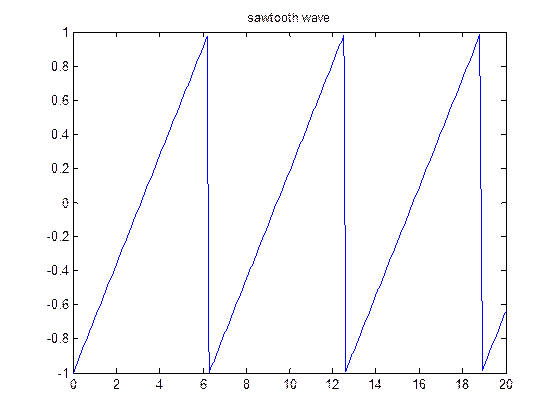CONCLUSION: